top of page
##### CLASSIC PRIZES TO DATE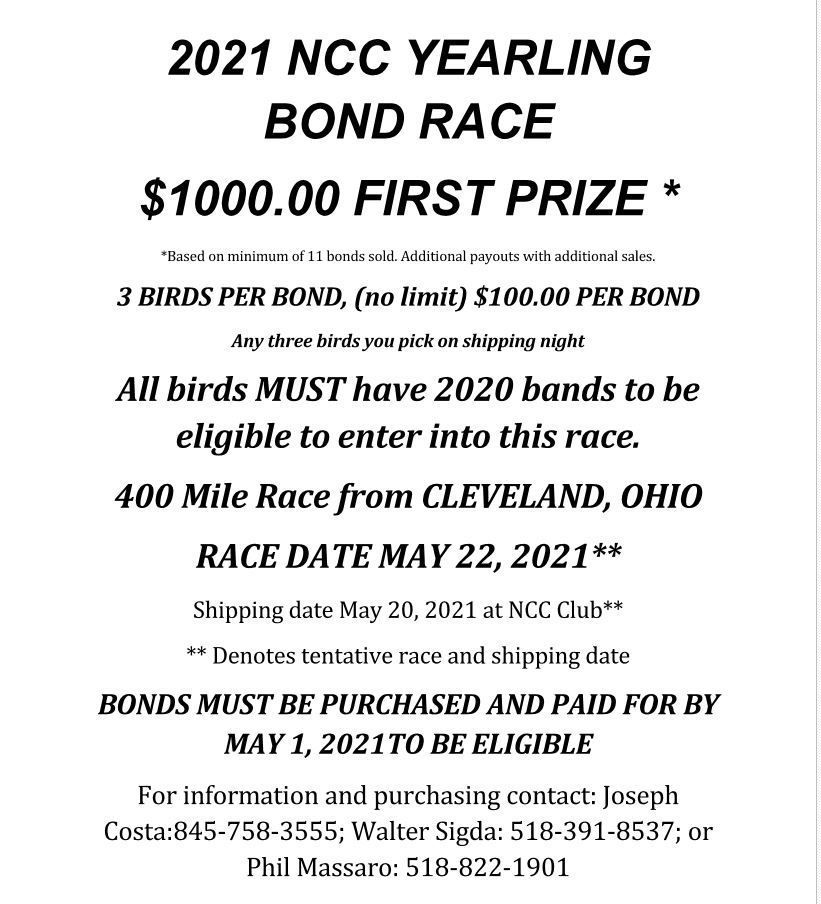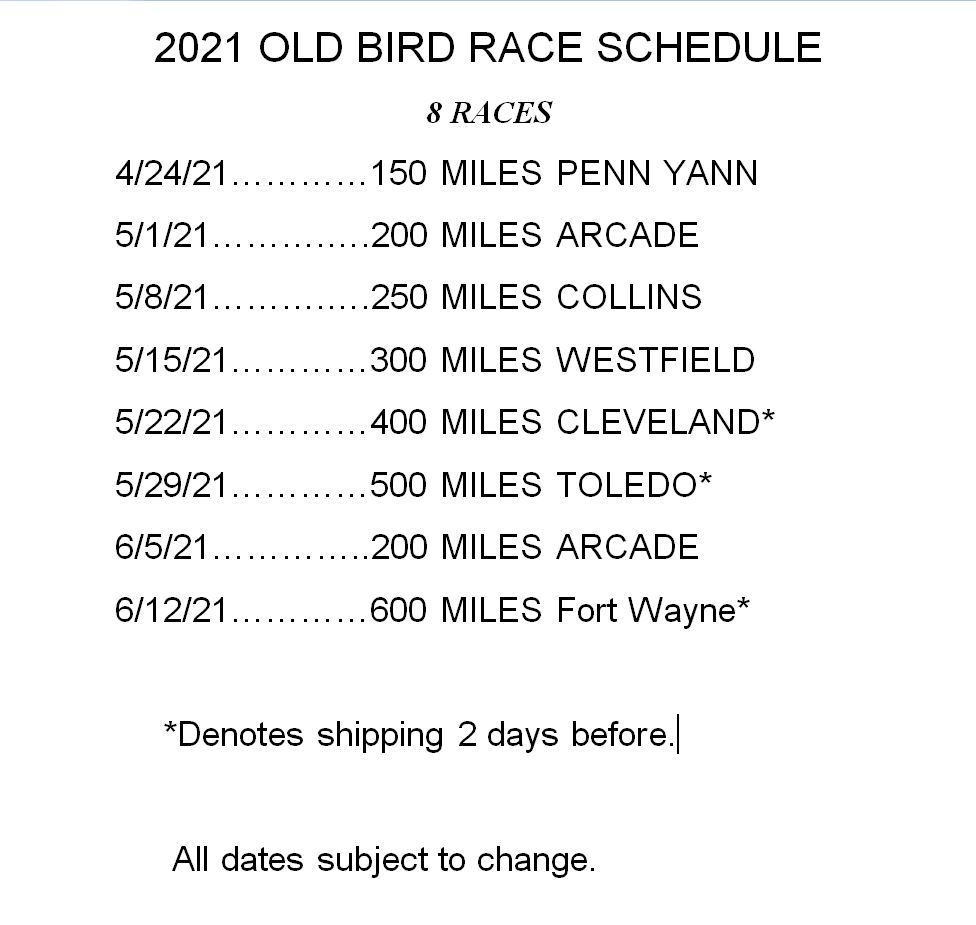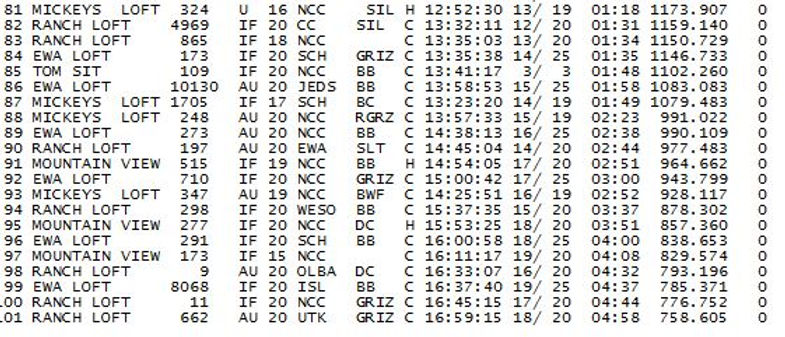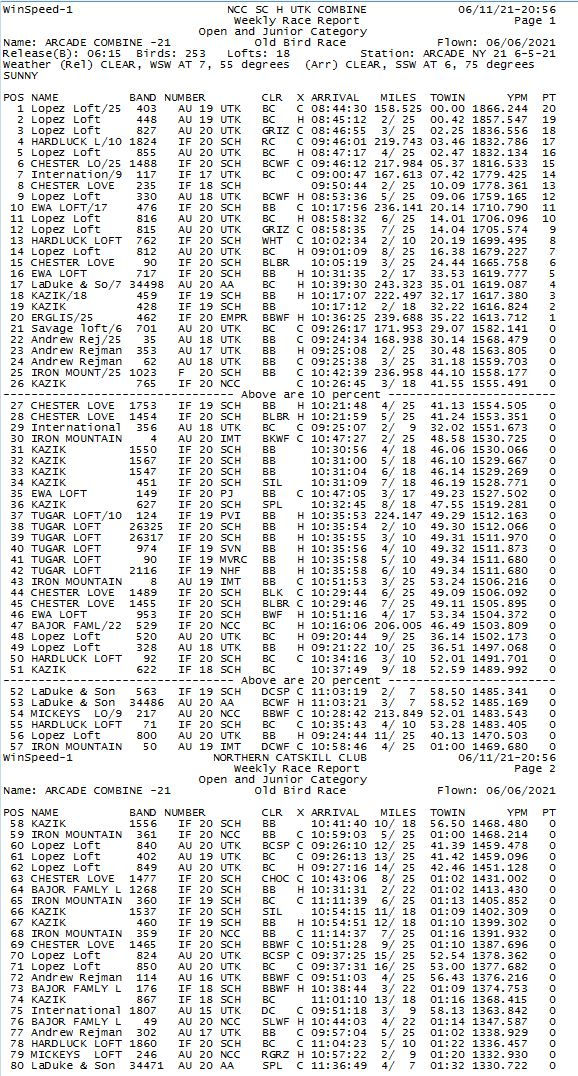# 1st = 5,000 Points 2nd = 2,500 Points 3rd = 1,500 points 4th = 1,000 points 5th = 900 Points 6th = 600 Points 7th = 500 points 8th = 450 Points 9th = 350 Points 10th = 250 Points 11th = 200 Points 12th = 200 Points 13th = 200 Points 14th = 150 Points 15th =100 Points

##### 2021 YOUNG BIRD  RESULTS##### 2021 CLASSIC RESULTS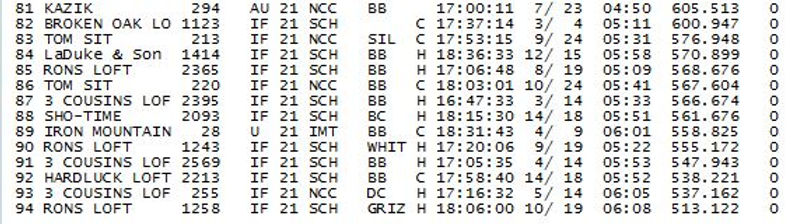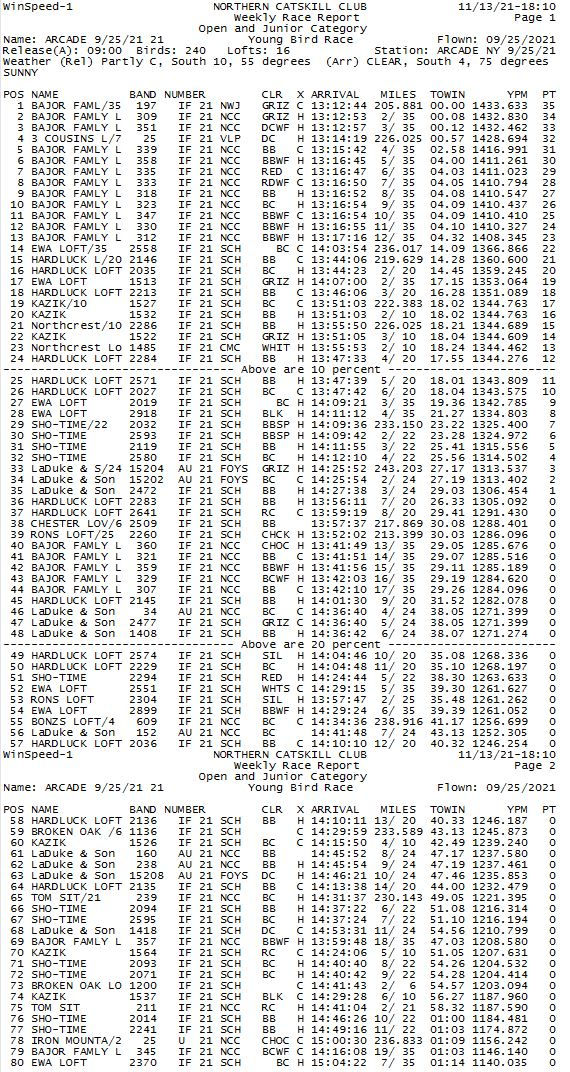bottom of page